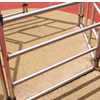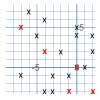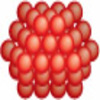Resources tagged with: Visualising

Filter by: Content type:
Age range:
Challenge level:

There are 179 results

Broad Topics > Thinking Mathematically > VisualisingAge 11 to 14Challenge Level

Four rods, two of length a and two of length b, are linked to form a kite. The linkage is moveable so that the angles change. What is the maximum area of the kite?Rati-o

Age 11 to 14Challenge Level

Points P, Q, R and S each divide the sides AB, BC, CD and DA respectively in the ratio of 2 : 1. Join the points. What is the area of the parallelogram PQRS in relation to the original rectangle?Star Gazing

Age 14 to 16Challenge Level

Find the ratio of the outer shaded area to the inner area for a six pointed star and an eight pointed star.Dotty Triangles

Age 11 to 14Challenge Level

Imagine an infinitely large sheet of square dotty paper on which you can draw triangles of any size you wish (providing each vertex is on a dot). What areas is it/is it not possible to draw?Age 14 to 16Challenge Level

In this problem we are faced with an apparently easy area problem, but it has gone horribly wrong! What happened?Triangular Tantaliser

Age 11 to 14Challenge Level

Draw all the possible distinct triangles on a 4 x 4 dotty grid. Convince me that you have all possible triangles.The Farmers' Field Boundary

Age 11 to 14Challenge Level

The farmers want to redraw their field boundary but keep the area the same. Can you advise them?Rolling Around

Age 11 to 14Challenge Level

A circle rolls around the outside edge of a square so that its circumference always touches the edge of the square. Can you describe the locus of the centre of the circle?Tied Up

Age 14 to 16 ShortChallenge Level

How much of the field can the animals graze?Trice

Age 11 to 14Challenge Level

ABCDEFGH is a 3 by 3 by 3 cube. Point P is 1/3 along AB (that is AP : PB = 1 : 2), point Q is 1/3 along GH and point R is 1/3 along ED. What is the area of the triangle PQR?Isosceles Triangles

Age 11 to 14Challenge Level

Draw some isosceles triangles with an area of $9$cm$^2$ and a vertex at (20,20). If all the vertices must have whole number coordinates, how many is it possible to draw?Age 14 to 16Challenge Level

Four rods are hinged at their ends to form a convex quadrilateral. Investigate the different shapes that the quadrilateral can take. Be patient this problem may be slow to load.The Old Goats

Age 11 to 14Challenge Level

A rectangular field has two posts with a ring on top of each post. There are two quarrelsome goats and plenty of ropes which you can tie to their collars. How can you secure them so they can't. . . .Like a Circle in a Spiral

Age 7 to 16Challenge Level

A cheap and simple toy with lots of mathematics. Can you interpret the images that are produced? Can you predict the pattern that will be produced using different wheels?Shear Magic

Age 11 to 14Challenge Level

Explore the area of families of parallelograms and triangles. Can you find rules to work out the areas?Coke Machine

Age 14 to 16Challenge Level

The coke machine in college takes 50 pence pieces. It also takes a certain foreign coin of traditional design...Around and Back

Age 14 to 16Challenge Level

A cyclist and a runner start off simultaneously around a race track each going at a constant speed. The cyclist goes all the way around and then catches up with the runner. He then instantly turns. . . .An Unusual Shape

Age 11 to 14Challenge Level

Can you maximise the area available to a grazing goat?A Tilted Square

Age 14 to 16Challenge Level

The opposite vertices of a square have coordinates (a,b) and (c,d). What are the coordinates of the other vertices?Zooming in on the Squares

Age 7 to 14

Start with a large square, join the midpoints of its sides, you'll see four right angled triangles. Remove these triangles, a second square is left. Repeat the operation. What happens?Coloured Edges

Age 11 to 14Challenge Level

The whole set of tiles is used to make a square. This has a green and blue border. There are no green or blue tiles anywhere in the square except on this border. How many tiles are there in the set?Convex Polygons

Age 11 to 14Challenge Level

Show that among the interior angles of a convex polygon there cannot be more than three acute angles.Tilting Triangles

Age 14 to 16Challenge Level

A right-angled isosceles triangle is rotated about the centre point of a square. What can you say about the area of the part of the square covered by the triangle as it rotates?Cutting a Cube

Age 11 to 14Challenge Level

A half-cube is cut into two pieces by a plane through the long diagonal and at right angles to it. Can you draw a net of these pieces? Are they identical?Playground Snapshot

Age 7 to 14Challenge Level

The image in this problem is part of a piece of equipment found in the playground of a school. How would you describe it to someone over the phone?Contact

Age 14 to 16Challenge Level

A circular plate rolls in contact with the sides of a rectangular tray. How much of its circumference comes into contact with the sides of the tray when it rolls around one circuit?Tetra Square

Age 14 to 16Challenge Level

ABCD is a regular tetrahedron and the points P, Q, R and S are the midpoints of the edges AB, BD, CD and CA. Prove that PQRS is a square.One and Three

Age 14 to 16Challenge Level

Two motorboats travelling up and down a lake at constant speeds leave opposite ends A and B at the same instant, passing each other, for the first time 600 metres from A, and on their return, 400. . . .Weighty Problem

Age 11 to 14Challenge Level

The diagram shows a very heavy kitchen cabinet. It cannot be lifted but it can be pivoted around a corner. The task is to move it, without sliding, in a series of turns about the corners so that it. . . .On the Edge

Age 11 to 14Challenge Level

If you move the tiles around, can you make squares with different coloured edges?Concrete Wheel

Age 11 to 14Challenge Level

A huge wheel is rolling past your window. What do you see?Something in Common

Age 14 to 16Challenge Level

A square of area 3 square units cannot be drawn on a 2D grid so that each of its vertices have integer coordinates, but can it be drawn on a 3D grid? Investigate squares that can be drawn.Ten Hidden Squares

Age 7 to 14Challenge Level

These points all mark the vertices (corners) of ten hidden squares. Can you find the 10 hidden squares?Efficient Cutting

Age 11 to 14Challenge Level

Use a single sheet of A4 paper and make a cylinder having the greatest possible volume. The cylinder must be closed off by a circle at each end.LOGO Challenge - Circles as Animals

Age 11 to 16Challenge Level

See if you can anticipate successive 'generations' of the two animals shown here.Eight Hidden Squares

Age 7 to 14Challenge Level

On the graph there are 28 marked points. These points all mark the vertices (corners) of eight hidden squares. Can you find the eight hidden squares?Efficient Packing

Age 14 to 16Challenge Level

How efficiently can you pack together disks?Packing 3D Shapes

Age 14 to 16Challenge Level

What 3D shapes occur in nature. How efficiently can you pack these shapes together?Speeding Boats

Age 14 to 16Challenge Level

Two boats travel up and down a lake. Can you picture where they will cross if you know how fast each boat is travelling?Christmas Chocolates

Age 11 to 14Challenge Level

How could Penny, Tom and Matthew work out how many chocolates there are in different sized boxes?Auditorium Steps

Age 7 to 14Challenge Level

What is the shape of wrapping paper that you would need to completely wrap this model?Constructing Triangles

Age 11 to 14Challenge Level

Generate three random numbers to determine the side lengths of a triangle. What triangles can you draw?Polygon Pictures

Age 11 to 14Challenge Level

Can you work out how these polygon pictures were drawn, and use that to figure out their angles?Cube Paths

Age 11 to 14Challenge Level

Given a 2 by 2 by 2 skeletal cube with one route `down' the cube. How many routes are there from A to B?Just Opposite

Age 14 to 16Challenge Level

A and C are the opposite vertices of a square ABCD, and have coordinates (a,b) and (c,d), respectively. What are the coordinates of the vertices B and D? What is the area of the square?Picture Story

Age 14 to 16Challenge Level

Can you see how this picture illustrates the formula for the sum of the first six cube numbers?Dissect

Age 11 to 14Challenge Level

What is the minimum number of squares a 13 by 13 square can be dissected into?Marbles in a Box

Age 11 to 16Challenge Level

How many winning lines can you make in a three-dimensional version of noughts and crosses?Counting Triangles

Age 11 to 14Challenge Level

Triangles are formed by joining the vertices of a skeletal cube. How many different types of triangle are there? How many triangles altogether?Natural Sum

Age 14 to 16Challenge Level

The picture illustrates the sum 1 + 2 + 3 + 4 = (4 x 5)/2. Prove the general formula for the sum of the first n natural numbers and the formula for the sum of the cubes of the first n natural. . . .